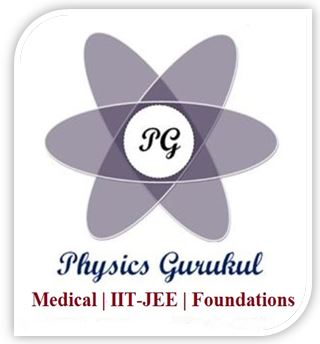# Class 11th Physics Ch-8 Gravitation Questions with Solutions

Question 1: The mass of two bodies are doubled and the distance is halved, how will the gravitational force change?

Question 2: If two masses are equal and made of same material, how will the force of attraction vary with separation?

Question 3: What is the height at which the value of g is the same as at a depth of R/2?

Question 4: A mass is dropped into a hole bored along the diameter of earth. What is the type of motion with the mass?

Question 5: If the earth stops rotating about its own axis, what is the effect on the value of g on the surface of earth?

Question 6: Can a pendulum undergo simple harmonic motion in a satellite?

Question 7: Two artificial satellites, one close to the surface and the other away are revolving around the earth. Which has larger speed?

Question 8: Which has a longer time period, a satellite revolving close or away from the surface of the earth?

Question 9: Will 1 kg sugar weigh more at poles or at the equator?

Question 10: A satellite revolving around earth loses height. How will its time period be changed?

Question 11: What is the amount of work done in bringing a mass from the surface of Earth on one side to a point diametrically opposite to the other side?

Answer Since gravitational potential difference is zero therefore the work done is zero.

Question 12: What would happen to an artificial satellite, if its orbital velocity is slightly decreased due to some defects in it?

Answer It will fall onto the Earth.

Question 13: What is 1 kg-wt?

Answer 1 Kg. wt = 9.8 newton.

Question 14: Define gravitational field intensity at any point? Write its S.I. units.

Answer Gravitational field intensity is defined as the force experienced per unit mass kept at that point. S.I. unit is N/Kg.

Question 15: What is the reason for absence of atmosphere in some planets ?

Answer very less escape velocity of 2.38 km/sec.

Question 16: Define potential difference between a pair of points in a gravitational field.

Question 17: Why does a person in a satellite feels weightlessness?

Answer Since no reaction is experienced by the person, he feels weightlessness.

Question 18: If the choice of origin is shifted what is the change in (i) Gravitational potential (ii) Potential energy?

(i) Remains dependent on the choice of origin.
(ii) Potential energy is independent since it depends only on separation.

Question 19: Can a satellite be in an orbit in a plane not passing through the earth’s centre? Explain your answer.

Answer The centripetal force required for the orbital motion of the satellite is provided by the gravitational force of attraction. Gravitational force is a central force, i.e., it passes through the centre of mass of the earth and the satellite. Hence the plane of orbit of the satellite has to pass through the earth’s centre.

Question 20: If earth be at one half its present distance from the sun, how many days will there be in a year?

Question 21: The masses M in the two figures are identical. Which of the two systems has the smaller gravitational potential energy ? Why ?

Answer The first system has the outer most masses closer to each other than the second system. Hence the gravitational P.E. of the second system is lesser than that of the first system.Announcements

Join our Online JEE Test Series for 499/- Only (Web + App) for 1 Year

Join our Online NEET Test Series for 499/- Only for 1 Year